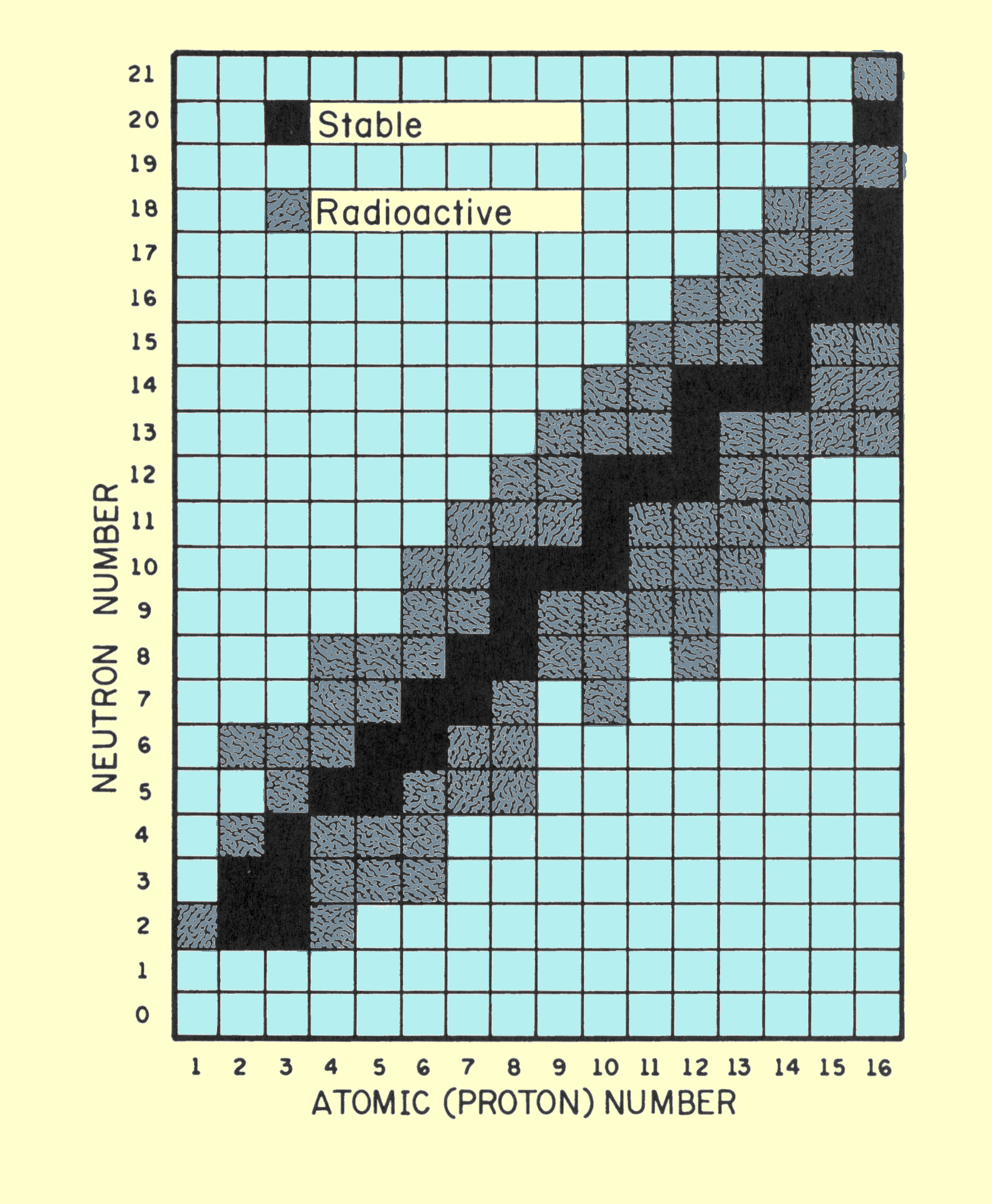# Why does oxygen-15 undergo positron decay?

May 29, 2015

Oxygen-15 undergoes positron decay because it has too few neutrons for the number of protons.

The principal factor that determines whether a nucleus is stable is the neutron to proton (n:p) ratio.

The diagram below plots the number of neutrons versus the number of protons in the first 15 elements.The black squares represent stable isotopes. They are called the band of stability for these elements .

For most of these elements, n:p = 1.

Oxygen-15 has 7 neutrons and 8 protons.

It is unstable because it is below the band of stability.

Its n:p ratio is 7:8 or 0.88:1.

It has too few neutrons for the number of protons, but it would become more stable if it could gain a neutron or lose a proton.

One way to do this is by positron (β⁺) decay.

""_1^1"p" → ""_0^1"n" + _1^0"e" + _0^0 ν_e

β⁺ decay accomplishes both of these.

It gets rid of a proton and forms a neutron (and a neutrino).

For oxygen-15, the reaction is

""_8^15"O" → _7^15"N" + _1^0"e" + _0^0 ν_e

Note that nitrogen-15 has 8 neutrons and 7 protons. Its n:p ratio is 1:1.1, and it is stable.